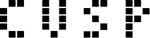# Iterative methods for sparse linear systems on GPU

### by Dr Nathan Bell

NVIDIA Research

This course will introduce students to iterative methods for solving sparse linear systems and how they are efficiently implemented on the GPU.  Students will gain intuition for the performance characteristics of sparse matrix operations and how to choose among sparse matrix representations.  We will show how generic parallel algorithms or “parallel primitives” can be used to manipulate sparse,  unstructured data sets and build complex algorithms.  A final lecture will summarize potential optimization techniques and methods for preconditioning iterative solvers to accelerate convergence.  A tentative course schedule follows below.

•Lesson 1: Sparse matrix representations and iterative solvers
• Lesson 2: Sparse matrix-vector multiplication (SpMV)
• Laboratory 1: Implementing an iterative solver on the GPU
• Lesson 3: Leveraging parallel primitives with Thrust
• Laboratory 2: Putting it all together
• Lesson 4 (30 mins): Future directions: optimization techniques and preconditioningLaboratory exercises will use the Thrust and CUSP libraries for CUDA.

### Student Requirements

The interested student should have:

• Understanding of standard (dense) linear algebra concepts
• Ability to write simple CUDA kernels (e.g. SAXPY)
• For this course, familiarity with C++ is beneficial but not necessary.